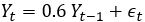# How to do it...

AR(1), MA(1), and ARMA(1,1) models are simulated first and the ACF and PACF plots are obtained:

1. First set the seed to the number 123. Produce three time series AR(1), MA(1), and ARMA(1,1):
```set.seed ( 123 ) t1 <- arima.sim ( list ( order = c ( 1 , 0 , 0 ), ar = 0.6 ), n = 100 ) t2 <- arima.sim ( list ( order = c ( 0 , 0 , 1 ), ma = - 0.2 ), n = 100 ) t3 <- arima.sim ( list ( order = c ( 1 , 0 , 1 ), ar = 0.6, ma= - 0.2 ), n = 100 ) tail (t1); tail (t2); tail (t3) #output suppressed
```

The underlying AR(1) model for t1 isand similarly for t2 and t3, the associated underlying models are:

1. Obtain the ACF and PACF plots of

Get Practical Data Science Cookbook - Second Edition now with the O’Reilly learning platform.

O’Reilly members experience books, live events, courses curated by job role, and more from O’Reilly and nearly 200 top publishers.